# 3.32. One mole of an ideal gas, initially at 30°C and 1 bar, is changed to 130°C and 10 bar by three different mechanic...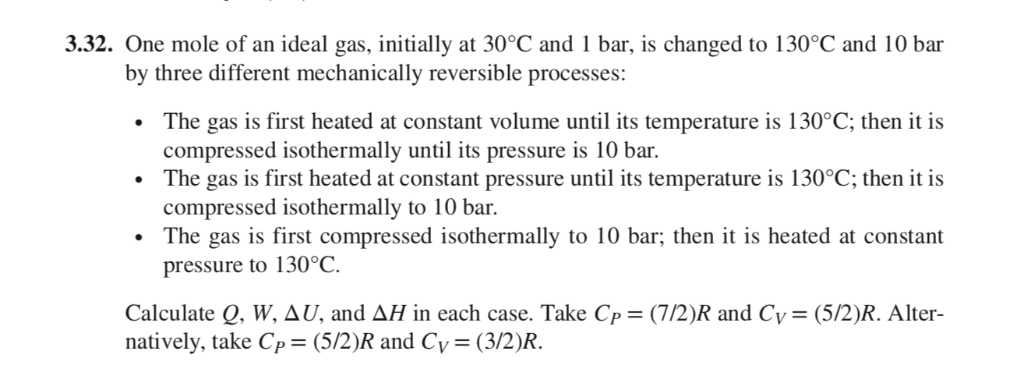3.32. One mole of an ideal gas, initially at 30°C and 1 bar, is changed to 130°C and 10 bar by three different mechanically reversible processes: The gas is first heated at constant volume until its temperature is 130°C; then it is compressed isothermally until its pressure is 10 bar The gas is first heated at constant pressure until its temperature is 130°C; then it is compressed isothermally to 10 bar The gas is first compressed isothermally to 10 bar; then it is heated at constant pressure to 130°C Calculate Q, W, AU, and AH in each case. Take Cp = (7/2)R and Cy = (5/2)R. Alter- natively, take Cp= (5/2)R_and Cy= (3/2)R.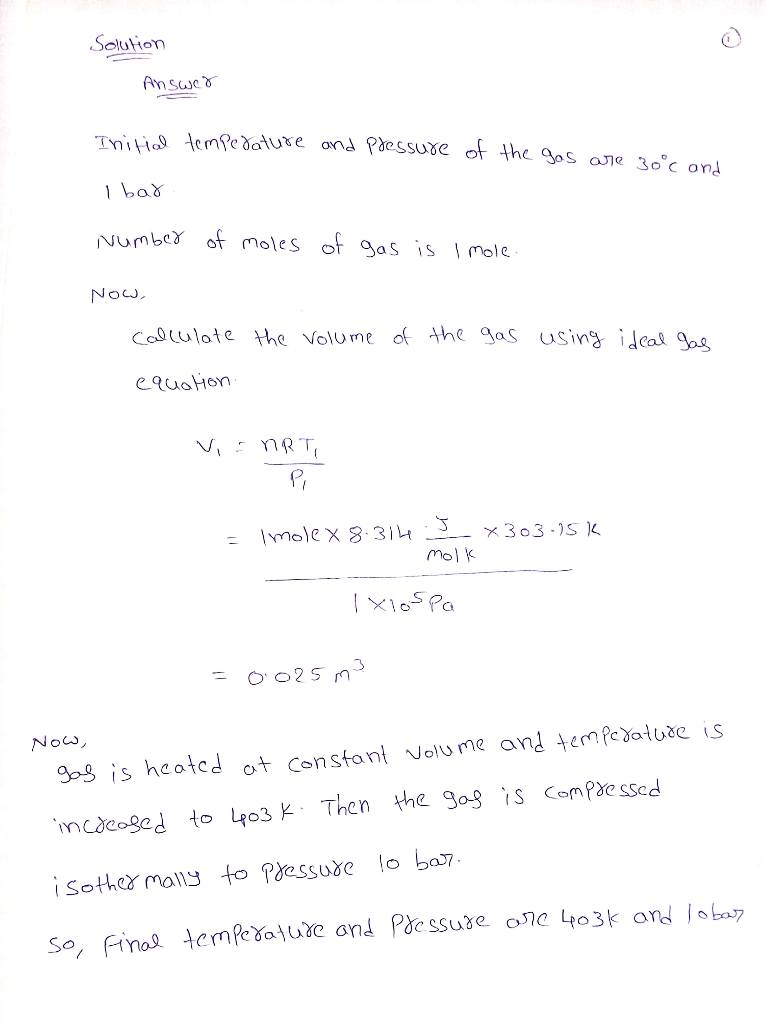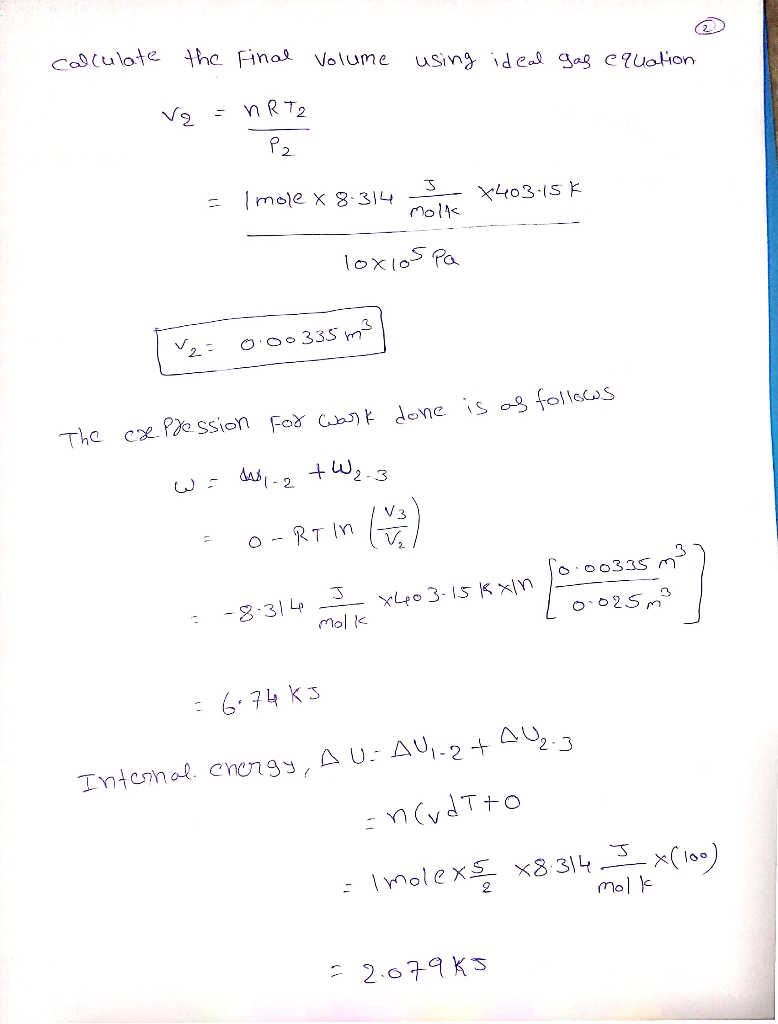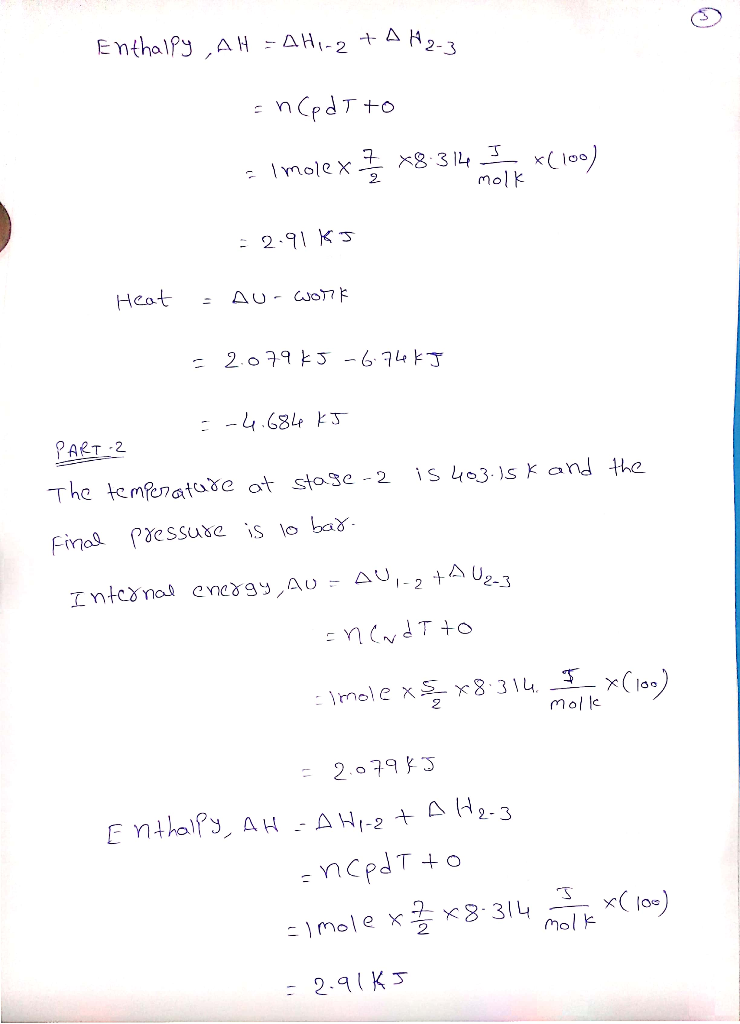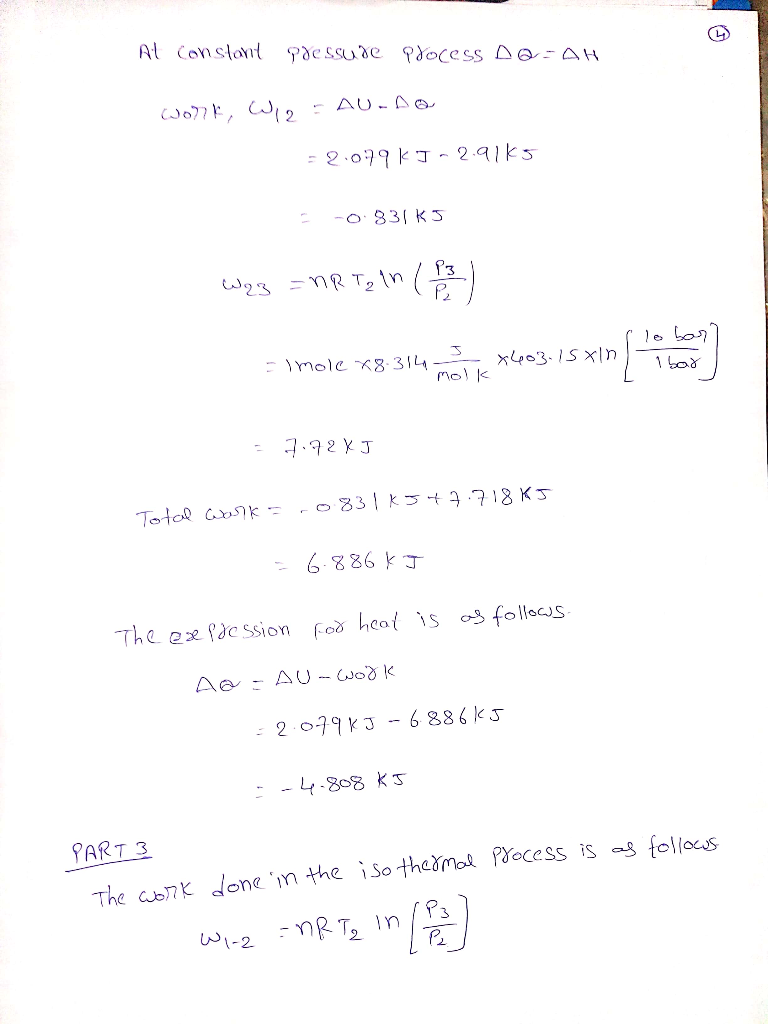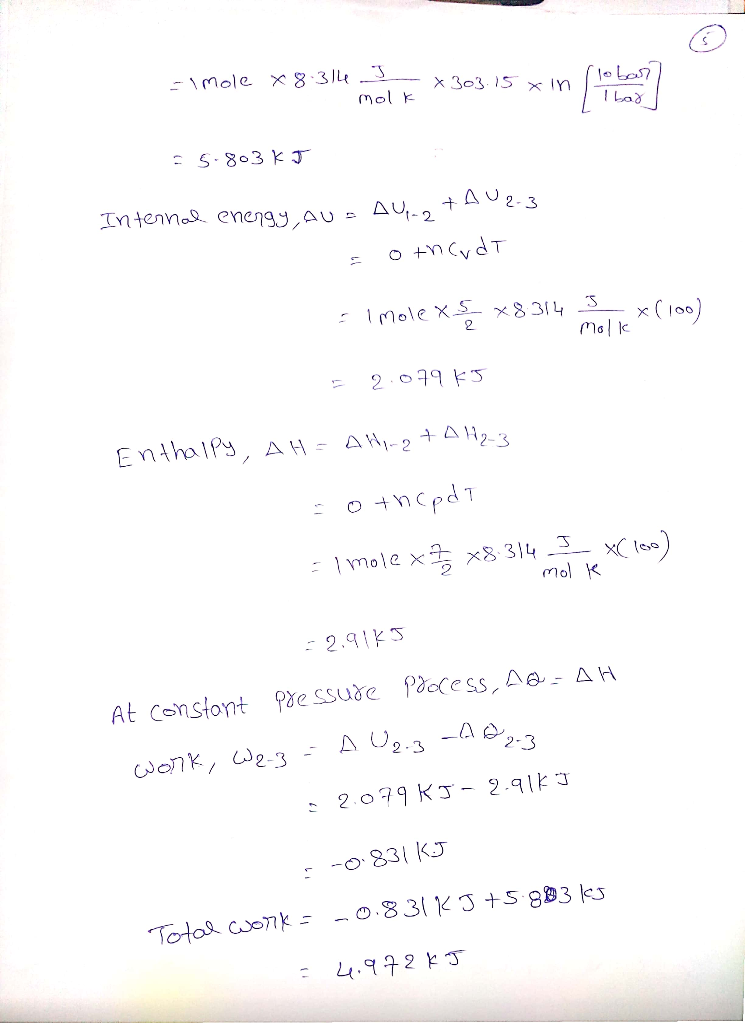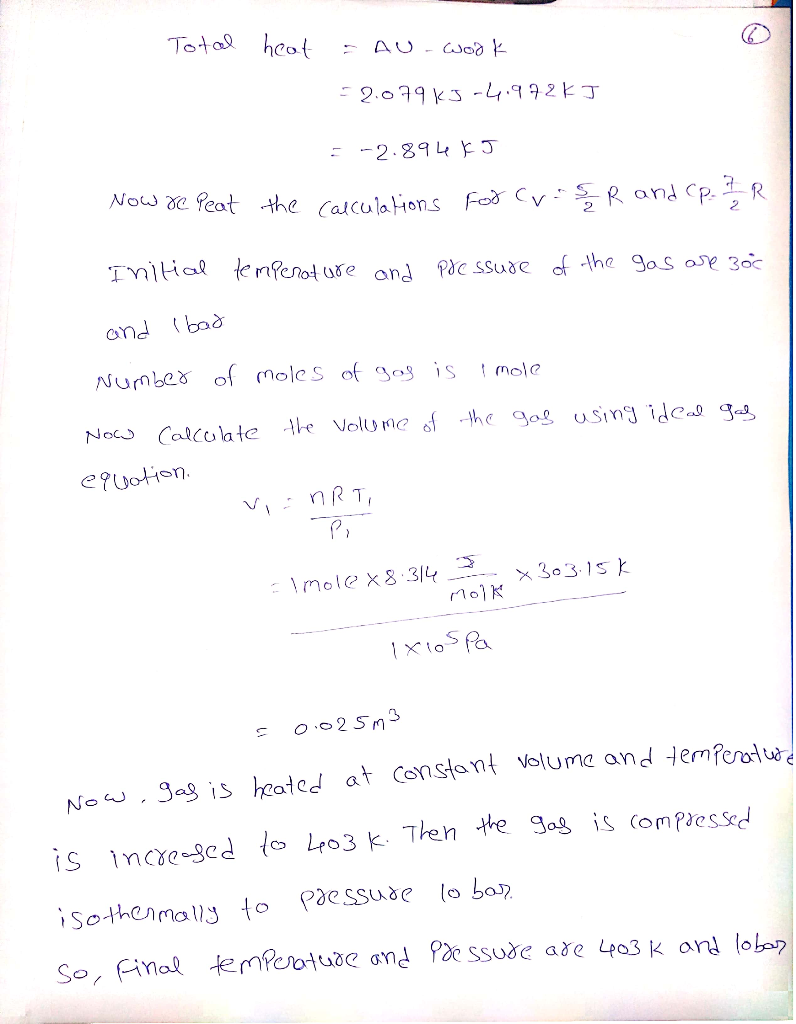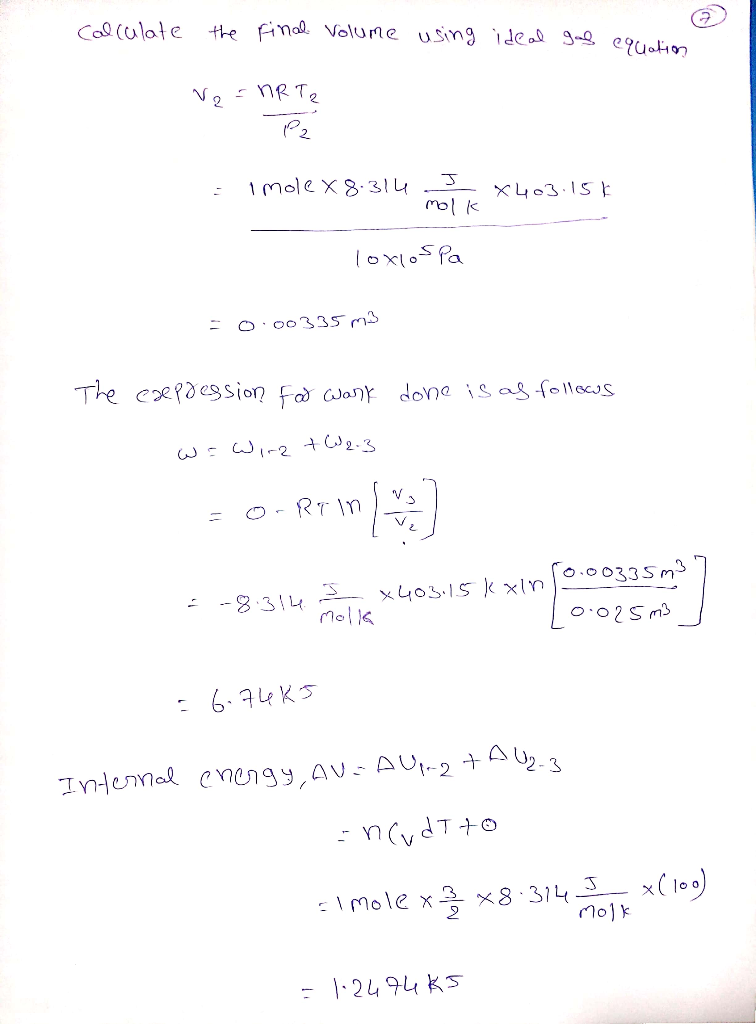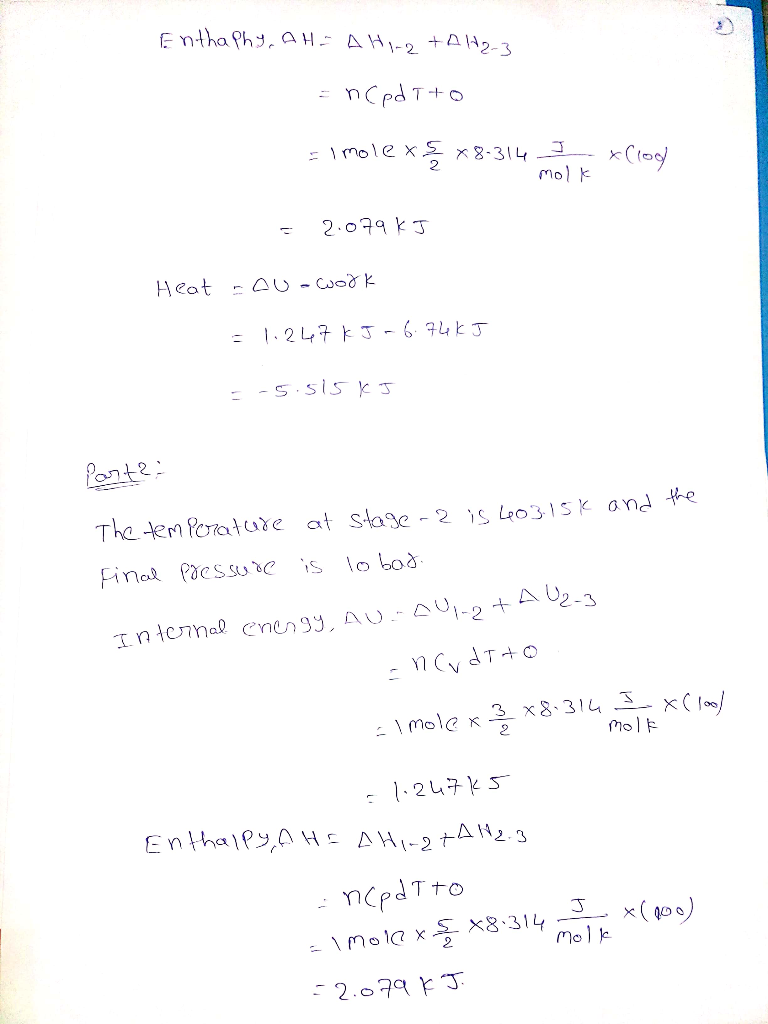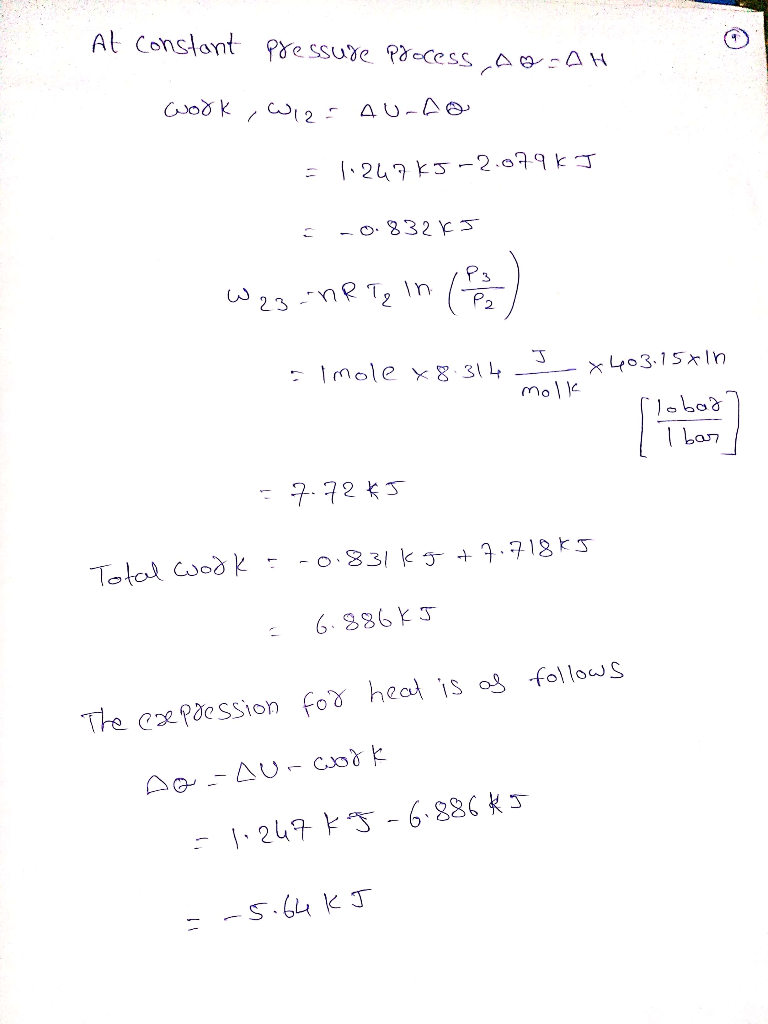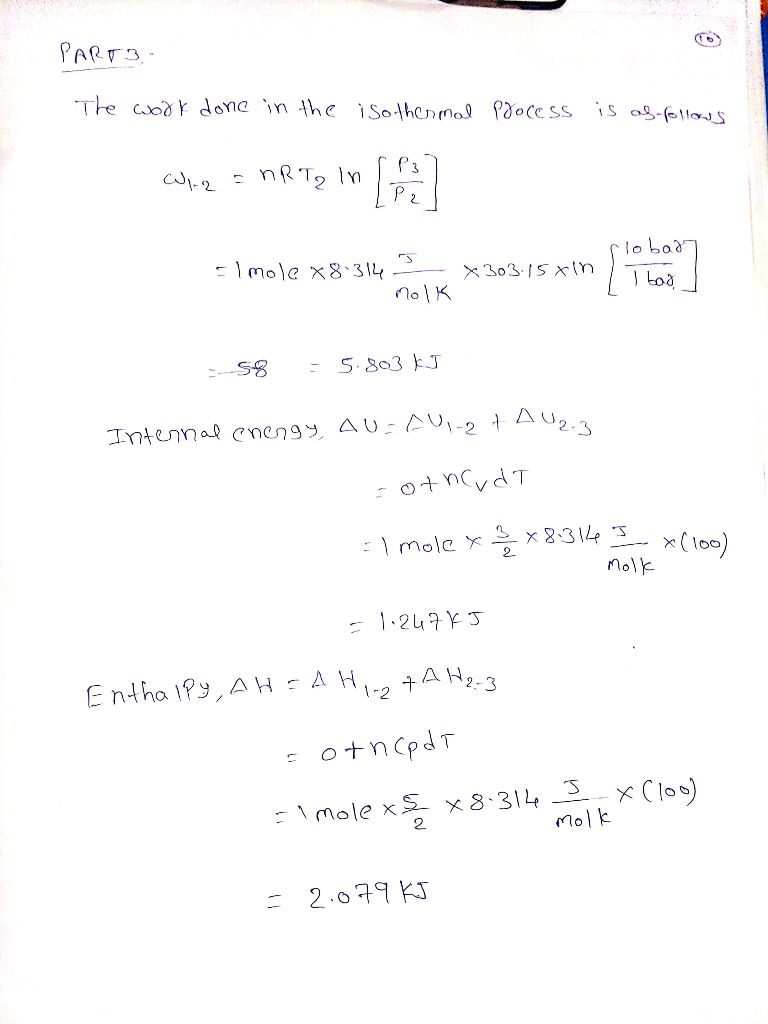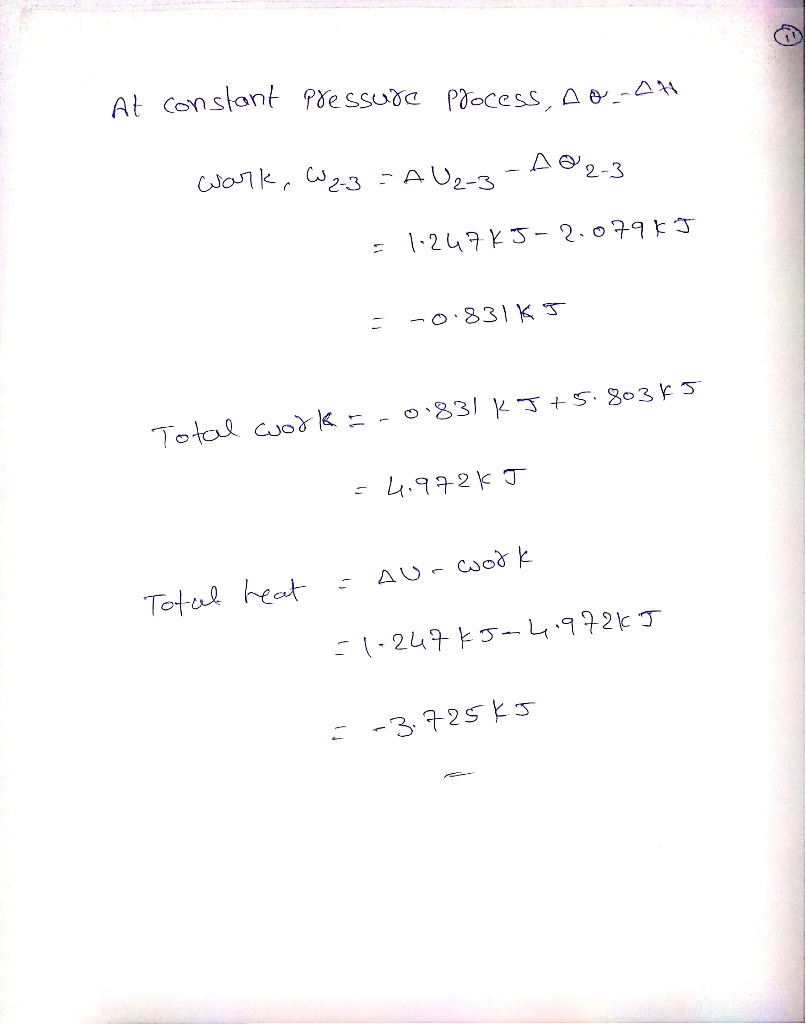##### Add Answer of: 3.32. One mole of an ideal gas, initially at 30°C and 1 bar, is changed to 130°C and 10 bar by three different mechanic...
Similar Homework Help Questions
• ### thermodynamics

One mole of an ideal gas, initially at 30 ºC and 1 bar undergoes the following mechanically reversible changes. It is compressed isothermally to a point such that whenit is heated at a constant volume to 120 ºC its final pressure is 12 bar. Calculate Q, W, ?U, ?H for the process. Assume CP = 7/2 R and CV = 5/2 R

• ### **PLEASE ANSWER ALL SUB-QUESTIONS AND EXPLAIN STEP BY STEP. THANK YOU!** QUESTION 6 One mole of an ideal gas is compres...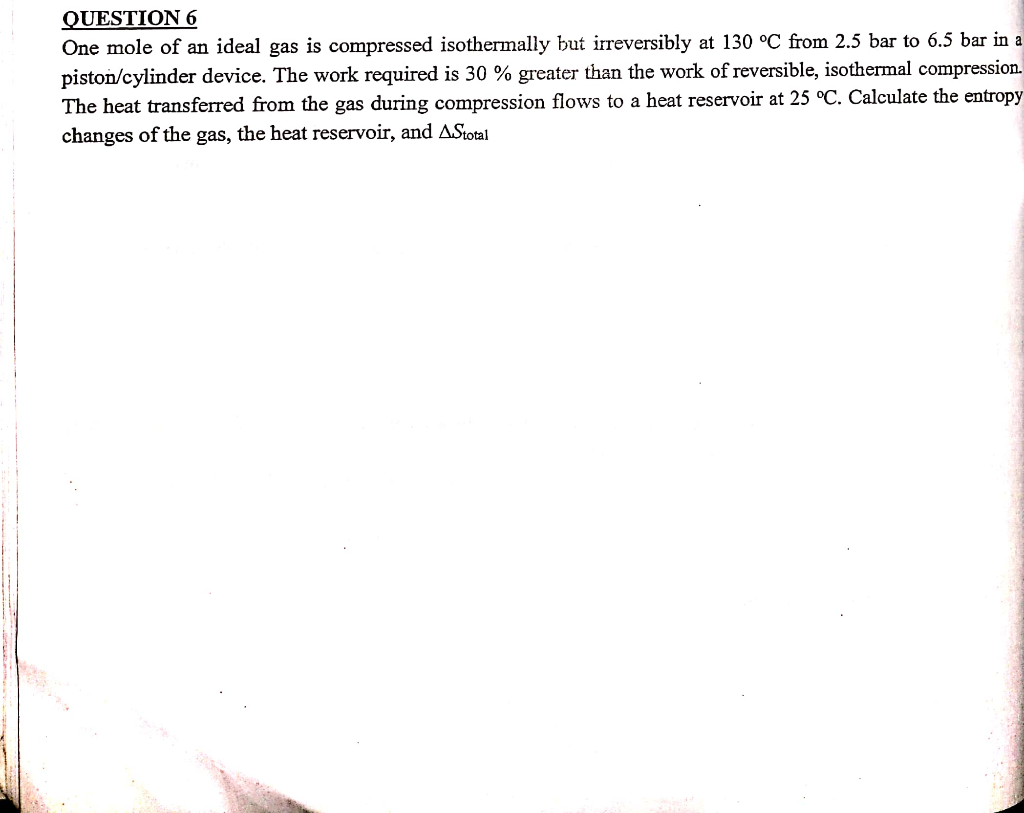**PLEASE ANSWER ALL SUB-QUESTIONS AND EXPLAIN STEP BY STEP. THANK YOU!** QUESTION 6 One mole of an ideal gas is compressed isothermally but irreversibly at 130 oC from 2.5 bar to 6.5 bar in a piston/cylinder device. The work required is 30 % greater than the work of reversible, isothermal compression. The heat transferred from the gas during compression flows to a heat reservoir at 25 °C. Calculate the entropy changes of the gas, the heat reservoir, and AStotal QUESTION...

• ### thermodynamics problem

////Please explain in great detail every step and every symbol and formula used so I may be able to//// understand the steps and do them on my own. Thanks, eddie.One mole of an ideal gas, initially at 20 degrees C and 1 bar, undergoes the following mechanically reversible changes. It is compressed isothermally to a pointsuch that when it is heated at constant volume to 100 degrees C it's final pressure is 10 bar. Calculate Q, W, change in U,...

• ### One mole of oxygen gas is at a pressure of 5.10 atm and a temperature of 32.0°C

One mole of oxygen gas is at a pressure of 5.10 atm and a temperature of 32.0°C. (a) If the gas is heated at constant volume until the pressure triples, what is the final temperature?

• ### One mole of oxygen gas is at a pressure of 5.55 atm and a temperature of 23.0°C.

One mole of oxygen gas is at a pressure of 5.55 atm and a temperature of 23.0°C.(a) If the gas is heated at constant volume until the pressure triples, what is the final temperature?(b) If the gas is heated so that both the pressure and volume are doubled, what is the final temperature?

• ### If an ideal gas initially at 0.6 bar and 1.5 L is compressed at constant temperature to a volume...

If an ideal gas initially at 0.6 bar and 1.5 L is compressed at constant temperature to a volume of 0.8 L, what is the final pressure of the compressed gas

• ### 4. (25pts) 20L nitrogen gas is compressed in a tank at 10 bar and 25°C. Calculate the maximum work (in joules) that can be obtained when the gas is allowed to expand reversibly to a pressure of 1...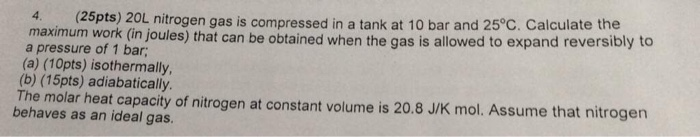4. (25pts) 20L nitrogen gas is compressed in a tank at 10 bar and 25°C. Calculate the maximum work (in joules) that can be obtained when the gas is allowed to expand reversibly to a pressure of 1 bar; (a) (10pts) isothermally (b) (15pts) adiabatically The molar heat apacity of nitrogen at constant volume is 20.8 J/K mol. Assume that nitrogen behaves as an ideal gas 4. (25pts) 20L nitrogen gas is compressed in a tank at 10 bar and...

• ### One gram-mole of an ideal gas

One gram-mole of an ideal gas initially occupies V1 = 5.0 L at P1 = 117 kPa, and then expands to V2 = 10.0 L at constant pressure.a) How much work was performed by the gas on its surroundings?b) How much heat is absorbed (+) or liberated (-)?c) What is the change in the internal energy of the gas?

• ### One mole of an ideal gas initially at a temperature of Ti = 4°C undergoes an expansion at a...

One mole of an ideal gas initially at a temperature of Ti = 4°C undergoes an expansion at a constant pressure of 1.00 atm, to four times its original volume.

• ### 3. A mole of perfect Gas initially at 101 325 pa is expanded from 22.4 L...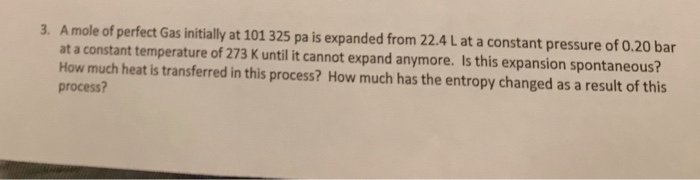3. A mole of perfect Gas initially at 101 325 pa is expanded from 22.4 L at a constant pressure of 0.20 bar at a constant temperature of 273 K until it cannot expand anymore. Is this expansion spontaneous? How much heat is transferred in this process? How much has the entropy changed as a result of this process?

Need Online Homework Help?